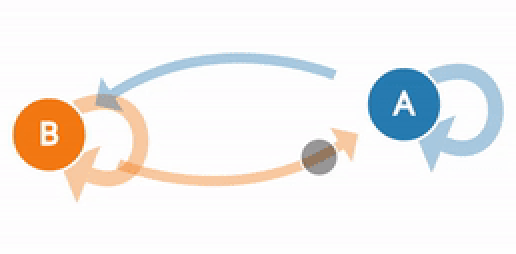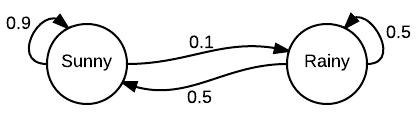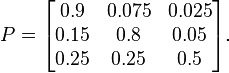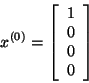Topics: Coronavirus | AI | Data Science | Deep Learning | Machine Learning | Python | R | Statistics

KDnuggets Home » News » 2018 » Mar » Tutorials, Overviews » Introduction to Markov Chains ( 18:n12 )

#Introduction to Markov Chains

What are Markov chains, when to use them, and how they workcomments

By Devin Soni, Computer Science Student(Generated from http://setosa.io/ev/markov-chains/)

Markov chains are a fairly common, and relatively simple, way to statistically model random processes. They have been used in many different domains, ranging from text generation to financial modeling. A popular example is r/SubredditSimulator, which uses Markov chains to automate the creation of content for an entire subreddit. Overall, Markov Chains are conceptually quite intuitive, and are very accessible in that they can be implemented without the use of any advanced statistical or mathematical concepts. They are a great way to start learning about probabilistic modeling and data science techniques.

Scenario

To begin, I will describe them with a very common example:

Imagine that there were two possible states for weather: sunny or cloudy. You can always directly observe the current weather state, and it is guaranteed to always be one of the two aforementioned states.

Now, you decide you want to be able to predict what the weather will be like tomorrow. Intuitively, you assume that there is an inherent transition in this process, in that the current weather has some bearing on what the next day’s weather will be. So, being the dedicated person that you are, you collect weather data over several years, and calculate that the chance of a sunny day occurring after a cloudy day is 0.25. You also note that, by extension, the chance of a cloudy day occurring after a cloudy day must be 0.75, since there are only two possible states.

You can now use this distribution to predict weather for days to come, based on what the current weather state is at the time.

This example illustrates many of the key concepts of a Markov chain. A Markov chain essentially consists of a set of transitions, which are determined by some probability distribution, that satisfy the Markov property.

Observe how in the example, the probability distribution is obtained solely by observing transitions from the current day to the next. This illustrates the Markov property, the unique characteristic of Markov processes that renders them memoryless. This typically leaves them unable to successfully produce sequences in which some underlying trend would be expected to occur. For example, while a Markov chain may be able to mimic the writing style of an author based on word frequencies, it would be unable to produce text that contains deep meaning or thematic significance since these are developed over much longer sequences of text. They therefore lack the ability to produce context-dependent content since they cannot take into account the full chain of prior states.A visualization of the weather example

The Model

Formally, a Markov chain is a probabilistic automaton. The probability distribution of state transitions is typically represented as the Markov chain’s transition matrixIf the Markov chain has N possible states, the matrix will be an N x N matrix, such that entry (I, J) is the probability of transitioning from state to state J. Additionally, the transition matrix must be a stochastic matrix, a matrix whose entries in each row must add up to exactly 1. This makes complete sense, since each row represents its own probability distribution.General view of a sample Markov chain, with states as circles, and edges as transitionsSample transition matrix with 3 possible states

Additionally, a Markov chain also has an initial state vector, represented as an N x 1 matrix (a vector), that describes the probability distribution of starting at each of the N possible states. Entry of the vector describes the probability of the chain beginning at state I.Initial State Vector with 4 possible states

These two entities are typically all that is needed to represent a Markov chain.

We now know how to obtain the chance of transitioning from one state to another, but how about finding the chance of that transition occurring over multiple steps? To formalize this, we now want to determine the probability of moving from state I to state J over M steps. As it turns out, this is actually very simple to find out. Given a transition matrix P, this can be determined by calculating the value of entry (I, J) of the matrix obtained by raising P to the power of M. For small values of M, this can easily be done by hand with repeated multiplication. However, for large values of M, if you are familiar with simple Linear Algebra, a more efficient way to raise a matrix to a power is to first diagonalize the matrix.

Conclusion

Now that you know the basics of Markov chains, you should now be able to easily implement them in a language of your choice. If coding is not your forte, there are also many more advanced properties of Markov chains and Markov processes to dive into. In my opinion, the natural progression along the theory route would be toward Hidden Markov Processes or MCMC. Simple Markov chains are the building blocks of other, more sophisticated, modeling techniques, so with this knowledge, you can now move onto various techniques within topics such as belief modeling and sampling.

Bio: Devin Soni is a computer science student interested in machine learning and data science. He will be a software engineering intern at Airbnb in 2018. He can be reached via LinkedIn.

Original. Reposted with permission.

Related:Get KDnuggets, a leading newsletter on AI, Data Science, and Machine Learning